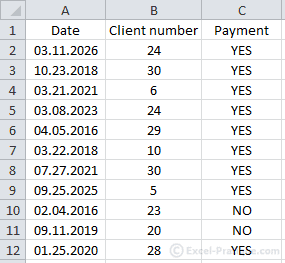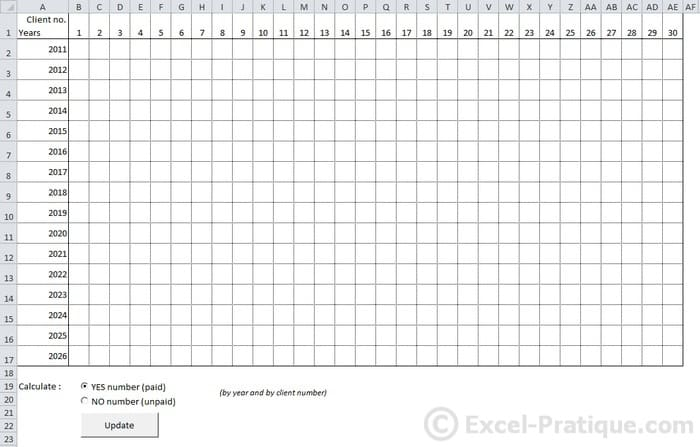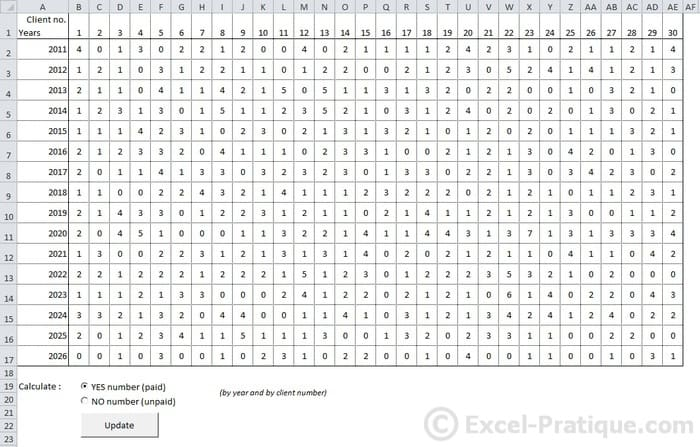# VBA Course: Using Arrays (exercise)

To practice using arrays, you will create your own version of the macro that we used to demonstrate the speed advantages of arrays, step by step ...

Here is the starting point of this exercise (you will see that the data set has been reduced to 1000 rows):Goal of the exercise: the procedure should process the data in the data set using a loop and count the number of "YES" or "NO" responses for each year and for each client number (either "YES" or "NO", depending on user selection) and enter this count in a specified cell on the worksheet.Complete the following macro to store the data set from worksheet "DS" in an array:

```Copy```Sub actualize()
Dim last_row As Integer

'Last row of the data set
'...

'Storing the data set in a dynamic array
Dim array_db()
'...

End Sub``````

.
.
.
.
.
.
.
.
.
.
.
.
.
.
.
.
.
.
.
.
.
.
.
.
.
.
.
.
.
.

Here is one solution:

```Copy```Sub actualize()
Dim last_row As Integer

'Last row of the database
last_row = Sheets("DS").Range("A1").End(xlDown).Row

'Storing the data set in a dynamic array
Dim array_db()
ReDim array_db(last_row - 2, 2)

For row_number = 2 To last_row
array_db(row_number - 2, 0) = Sheets("DS").Range("A" & row_number)
array_db(row_number - 2, 1) = Sheets("DS").Range("B" & row_number)
array_db(row_number - 2, 2) = Sheets("DS").Range("C" & row_number)
Next
End Sub``````

This essentially repeats what we did on the previous page ...

But now we need to modify the macro by adding the following actions:

• Determine the user's choice ("YES" or "NO")
• Calculate the number of "YES" or "NO" responses in the data set in order to determin the size of the array (Redim)
• Store only the rows of the data set that contain "YES" or "NO" responses in the array (this means that there's no need to store the data from the 3rd column)

.
.
.
.
.
.
.
.
.
.
.
.
.
.
.
.
.
.
.
.
.
.
.
.
.
.
.
.
.
.

Here is one solution:

```Copy```Sub actualize()
Dim last_row As Integer, search_value As String, insert_row As Integer, value_yes_no As String, rows_number As Integer

'Last row of the data set
last_row = Sheets("DS").Range("A1").End(xlDown).Row

'Search value (YES or NO)
If Sheets("RES").OptionButton_yes.Value = True Then
search_value = "YES"
Else
search_value = "NO"
End If

'Number of YES or NO responses
rows_number = WorksheetFunction.CountIf(Sheets("DS").Range("C2:C" & last_row), search_value)

'Storing the data set in the array
Dim array_db()
ReDim array_db(rows_number - 1, 1)

insert_row = 0

For row_number = 2 To last_row
value_yes_no = Sheets("DS").Range("C" & row_number)
If value_yes_no = search_value Then
array_db(insert_row, 0) = Sheets("DS").Range("A" & row_number)
array_db(insert_row, 1) = Sheets("DS").Range("B" & row_number)
insert_row = insert_row + 1
End If
Next
End Sub``````

The user's search choice is determined at the beginning of the procedure by the following code:

```Copy```'Search value (YES or NO)
If Sheets("RES").OptionButton_yes.Value = True Then
search_value = "YES"
Else
search_value = "NO"
End If``````

We are using the CountIF function to determine the number of YES or NO responses:

```Copy```'Number of YES or NO responses
rows_number = WorksheetFunction.CountIf(Sheets("DS").Range("C2:C" & last_row), search_value)``````

The size of the array has been adjusted to fit the number of YES or NO responses and reduced to two columns:

`Copy`ReDim array_db(rows_number - 1, 1)``

The data will now be stored in the array when its 3rd column corresponds to the user's search choice:

```Copy```'Insertion number in the array
insert_row = 0

'Processing the data set
For row_number = 2 To last_row
'Value of column C (YES or NO)
value_yes_no = Sheets("DS").Range("C" & row_number)
'If the value corresponds to the user's search choice, the row is stored in the array
If value_yes_no = search_value Then
'Storing the value of column A
array_db(insert_row, 0) = Sheets("DS").Range("A" & row_number)
'Storing the value of column B
array_db(insert_row, 1) = Sheets("DS").Range("B" & row_number)
'One row has been stored => the insertion number in the array is incremented by 1
insert_row = insert_row + 1
End If
Next``````

The array contains only the data that we are interested in.

All that we have left to do is to:

• Process each element of the table on the "RES" worksheet using 2 loops (this is the same idea as the Checkerboard exercise)
• And insert the total number of entries on this worksheet for a year by client number for each cell

.
.
.
.
.
.
.
.
.
.
.
.
.
.
.
.
.
.
.
.
.
.
.
.
.
.
.
.
.
.

Here is a solution:

```Copy```'Count of "YES"/"NO" responses
For no_years = 2011 To 2026
For no_client = 1 To 30
counter = 0
For i = 0 To UBound(array_db)
If Year(array_db(i, 0)) = no_years And array_db(i, 1) = no_client Then
counter = counter + 1
End If
Next
Cells(no_years - 2009, no_client + 1) = counter
Next
Next``````

The solution with commentaries that explain it in detail:

```Copy```'A loop for each row
For no_years = 2011 To 2026
'A loop for each column
For no_client = 1 To 30
'Counter re-initialized
counter = 0
'Processing the array
For i = 0 To UBound(array_db)
'Verify that the row in the table match the year and client number
If Year(array_db(i, 0)) = no_years And array_db(i, 1) = no_client Then
'If year and client number match, the counter is incremented by 1
counter = counter + 1
End If
Next
'After processing the array, the total is entered in the appropriate cell
Cells(no_years - 2009, no_client + 1) = counter
Next
Next``````

And finally, the code of the entire macro:

```Copy```Sub actualize()
Dim last_row As Integer, search_value As String, insert_row As Integer, value_yes_no As String, rows_number As Integer, counter As Integer

'Deleting contents
Range("B2:AE17").ClearContents

'Last row in the data set
last_row = Sheets("DS").Range("A1").End(xlDown).Row

'Search value (YES ou NO)
If Sheets("RES").OptionButton_yes.Value = True Then
search_value = "YES"
Else
search_value = "NO"
End If

'Number of YES or NO responses
rows_number = WorksheetFunction.CountIf(Sheets("DS").Range("C2:C" & last_row), search_value)

'Storing the data set in the array
Dim array_db()
ReDim array_db(rows_number - 1, 1)

insert_row = 0

For row_number = 2 To last_row
value_yes_no = Sheets("DS").Range("C" & row_number)
If value_yes_no = search_value Then
array_db(insert_row, 0) = Sheets("DS").Range("A" & row_number)
array_db(insert_row, 1) = Sheets("DS").Range("B" & row_number)
insert_row = insert_row + 1
End If
Next

'Count of YES or NO responses
For no_years = 2011 To 2026
For no_client = 1 To 30
counter = 0
For i = 0 To UBound(array_db)
If Year(array_db(i, 0)) = no_years And array_db(i, 1) = no_client Then
counter = counter + 1
End If
Next
Cells(no_years - 2009, no_client + 1) = counter
Next
Next
End Sub``````